Get instant live expert help with Excel or Google Sheets“My Excelchat expert helped me in less than 20 minutes, saving me what would have been 5 hours of work!”

#### Post your problem and you’ll get expert help in seconds.

Your message must be at least 40 characters
Our professional experts are available now. Your privacy is guaranteed.

# How to Do Excel Data Validation Using the SUM Function

Data Validation in Excel allows you to create custom rules catered to your requirements. You can create a data validation using the SUM function to allow values, which added together, does not exceed a preset total. In this tutorial, you will learn how to create a data validation to only allow values that don’t exceed a set sum.

## Data Validation to Only Allow Values That Don’t Exceed a Set Sum

You can create a data validation rule to only allow values that don’t exceed a set sum using the SUM formula. The following example uses a monthly budget data set. Column A has the item names. To allow values in column B so that the total doesn’t exceed \$4,000:

1. Select cell B2. Drag the selection till B7 with your mouse.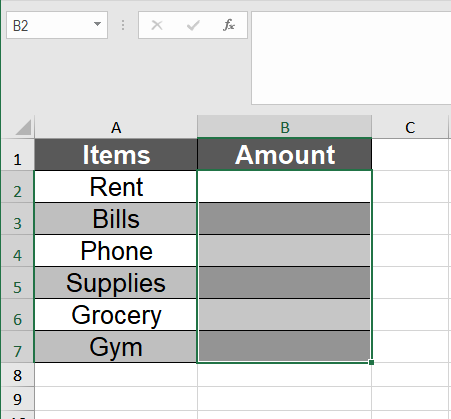2. Go to Data > Data Validation.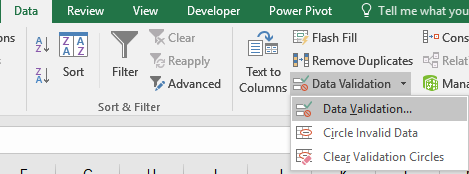3. From the drop-down in Allow, select Custom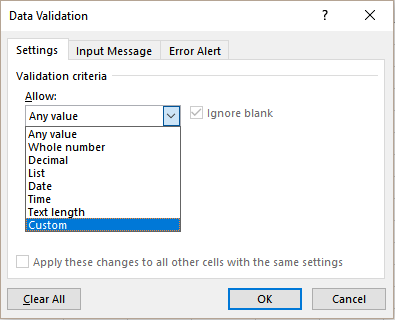4. In the Formula box, write the formula =`SUM(\$B\$2:\$B\$7) < 4000.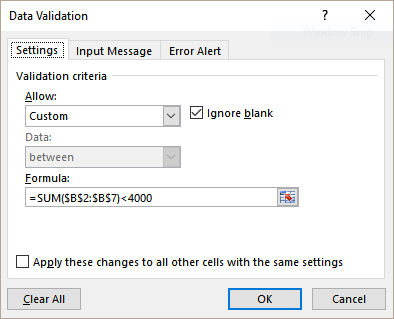`
5. Click OK.

This will only allow values in column B so that the total does not exceed \$4,000. The rule is triggered once a user adds or modifies a value. Here, the formula returns`=SUM(\$B\$2:\$B\$7) < 4000` FALSE as long as entries in B2:B7 totals to a value less than \$4,000. The sum covers an absolute reference in cells B2 to B7 to stop the reference from changing for each cell the validation is applied to. SUM is used to sum this fixed range and check if the total is less than or greater than \$4,000.

Once you enter a new number, the validation is triggered. As long as the total of the values in B2 to B7 is less than \$4,000, the validation passes. If any value makes the total to exceed \$4,000, validation fails and displays an error message. You can modify this and show your own custom message. To do this:

• After completing step 4, go to Error Alert.
• In the Error Message box, set the message to The current input causes the sum to exceed \$4,000. Please enter a smaller number.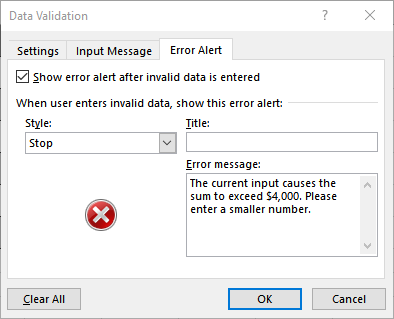• Click Ok.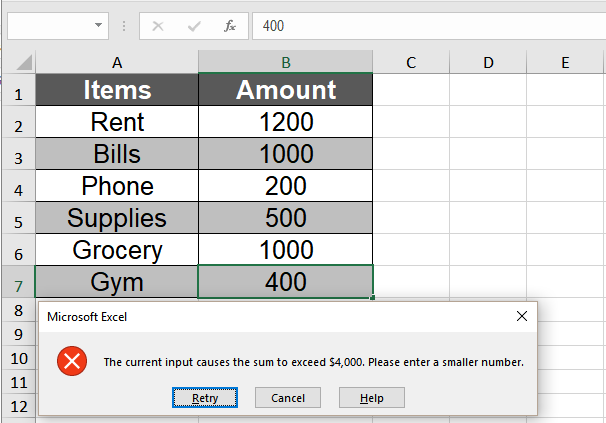Every time the validation fails, Excel will now show your custom error message.

Still need some help with Excel formatting or have other questions about Excel? Connect with a live Excel expert here for some 1 on 1 help. Your first session is always free.

### Did this post not answer your question? Get a solution from connecting with the expert.Another blog reader asked this question today on Excelchat:
Related blogs
Solution examplesI am trying to have a tab change color when the Sum of a row = 0. I am using code on the tab but it appears to be confused because the value of the given cell is not 0 it is "=SUM(G4:G17)"
Solved by S. F. in 41 minsI need a formula to pop at the upper left corner of a spreadsheet. If I enter the month "January," I want the column number sum of January =SUM(AB11:AB75) from another section on the same excel page to pop right below the "January" cell, and not display the formula expression, but see the \$100.
Solved by T. Q. in 40 minsCan't add (SUM) in imported numbers from bank account
Solved by F. C. in 40 minsI need a formula to combine D2 to D100 to add together a column of numbers, then take away the same amount on the same row when column E is filled. i.e. column D is a price of an item, so the formula must calculate the total, then when the item is sold an 'a' is marked next to the item in column E, the formula then must deduct this amount from the total
Solved by X. W. in 20 minsI would like to have a diagram in a new sheet, where the horizontal axis is the days, as they are in column DX. Each day shall show the sum of all unique leads of that day, and I would like to be able to check via a box of checkboxes, which facilities are shown, the facilities are in column BC.
Solved by I. A. in 45 mins## Subscribe to Excelchat.coAnother blog reader asked this question today on Excelchat: